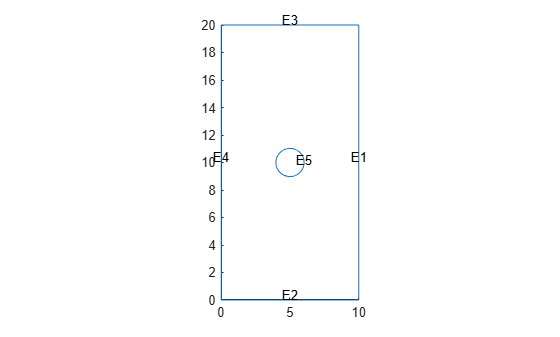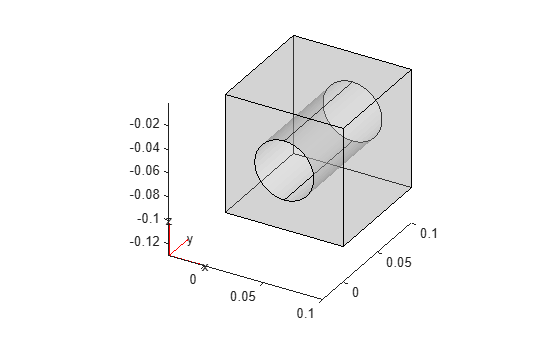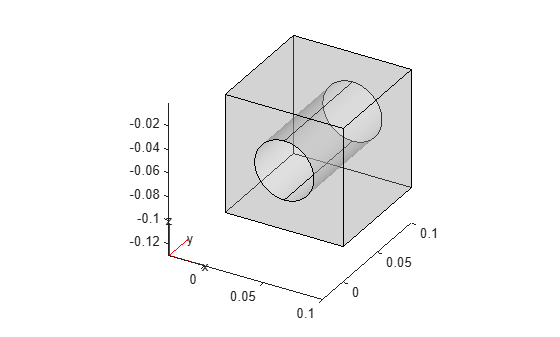# importGeometry

Import geometry from STL or STEP file

## Syntax

``gm = importGeometry(geometryfile)``
``gm = importGeometry(model,geometryfile)``
``importGeometry(model,___)``
``___ = importGeometry(___,"MaxRelativeDeviation",d)``

## Description

example

````gm = importGeometry(geometryfile)` creates a geometry object from the specified STL or STEP geometry file. A geometry imported from an STL file can be 3-D or planar. A geometry imported from a STEP file must be 3-D.```
````gm = importGeometry(model,geometryfile)` also includes the geometry in the `model` container.```

example

````importGeometry(model,___)` creates a geometry object from the specified STL or STEP geometry file and includes the geometry in the `model` container.```

example

````___ = importGeometry(___,"MaxRelativeDeviation",d)` lets you control the accuracy of the geometry import from a STEP file by specifying the relative sag. Use this syntax with any of the argument combinations from the previous syntaxes.```

## Examples

collapse all

Create a geometry object from an STL geometry file.

`gm = importGeometry("ForearmLink.stl");`

Plot the geometry.

`pdegplot(gm)`Import a planar STL geometry and include it in a PDE model. When importing a planar geometry, `importGeometry` converts it to a 2-D geometry by mapping it to the xy-plane.

Create a `PDEModel` container.

`model = createpde;`

Import a geometry into the container.

`importGeometry(model,"PlateHolePlanar.stl")`
```ans = DiscreteGeometry with properties: NumCells: 0 NumFaces: 1 NumEdges: 5 NumVertices: 5 Vertices: [5x3 double] ```

Plot the geometry with the edge labels.

`pdegplot(model,"EdgeLabels","on")`Create a geometry object from a STEP geometry file.

`gm = importGeometry("BlockWithHole.step");`

Plot the geometry.

`pdegplot(gm,"FaceAlpha",0.3)`Now import the same geometry while specifying the relative sag. You can use this parameter to control the accuracy of the geometry import.

`gm = importGeometry("BlockWithHole.step","MaxRelativeDeviation",10);`

Plot the geometry.

```figure pdegplot(gm,"FaceAlpha",0.3)```## Input Arguments

collapse all

Model container, specified as a `PDEModel` object, `ThermalModel` object, `StructuralModel` object, or `ElectromagneticModel` object.

Example: `model = createpde(3)`

Example: ```thermalmodel = createpde(thermal="steadystate")```

Example: ```structuralmodel = createpde(structural="static-solid")```

Example: ```emagmodel = createpde(electromagnetic="electrostatic")```

Path to STL or STEP file, specified as a string scalar or a character vector ending with the file extension `".stl"`, `".stp"`, or `".step"`. Use can also use the uppercase extensions `".STL"`, `".STP"` or `".STEP"`, or any combinations of uppercase and lowercase letters in these extensions.

Example: `"../geometries/Carburetor.stl"`

Data Types: `string` | `char`

Relative sag for importing a STEP geometry, specified as a number in the range [0.1, 10]. A relative sag is the ratio between the local absolute sag and the local mesh edge length. The absolute sag is the maximal gap between the mesh and the geometry.Example: ```gm = importGeometry("AngleBlock.step","MaxRelativeDeviation",5)```

Data Types: `double`

## Output Arguments

collapse all

Geometry, returned as a `DiscreteGeometry` object. See DiscreteGeometry Properties for details.

## Limitations

• `importGeometry` does not allow you to import a multidomain 2-D or 3-D geometry where subdomains have any common points. If the subdomains of the geometry have common points, the toolbox still treats these subdomains as disconnected, without any common interface between them. Each subdomain has its own mesh.

Because of this limitation, you cannot import nested 3-D geometries directly. As a workaround, you can import a mesh and then create a multidomain geometry from the mesh by using the `geometryFromMesh` function. See Multidomain Geometry Reconstructed from Mesh.

## Tips

• The STL format approximates the boundary of a CAD geometry by using a collection of triangles, and the `importGeometry` function reconstructs the faces and edges from this data. Reconstruction from STL data is not precise and can result in a loss of edges and, therefore, the merging of adjacent faces. Typically, lost edges are the edges between two adjacent faces meeting at a small angle, or smooth edges bounding blend surfaces. Usually, the loss of such edges does not affect the analysis workflow.• Because STL geometries are only approximations of the original CAD geometries, the areas and volumes of the STL and CAD geometries can differ.

## Version History

Introduced in R2015a

expand all